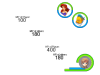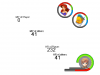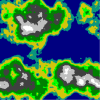# GMC ForumsGMC Archive - Programming

#### Nocturne

##### Friendly Tyrant
Forum Staff
This topic is for storing any posts that contain useful scripts and general code as well as any advanced programming knowledge that is still relevant to GameMaker Studio 2, culled from the defunct old GMC Archive. When posting please follow these simple rules:

1) All code must be 100% compatible with GameMaker Studio 2. If you wish to post code that is not compatible, then update it to be compatible before posting.

2) Please only make ONE post per topic, even if the source material comes from multiple posts. If the source material is too long, then we will permit consecutive posts, but try to keep it all as concise as possible (verbatim copy/paste is not required). Use code blocks and spoilers where appropriate to keep post size down.

3) Please give context for any code posted, explaining what it is and how it should be used. Do NOT just post blocks of code and leave it at that.

4) Please do NOT credit anyone for the posts. We are not allowed to have any personal information from the old forums due to GDPR guidelines.

Anything that is posted here that does not adhere to these rules will simply be removed. We want this to be a repository of the best of the knowledge contained in the old archive! Keep in mind that (as always) posts are subject to the usual forum rules and moderator discretion will be used to decide what is permitted and what is not.

IMPORTANT! If you have anything posted here from the old forums that belongs to you and you wish it removed, then please contact me VIA private message and we'll get it done. We do NOT want to archive any personal information or anything that may upset the original poster.

NOTE: If you wish to post anything from the Tutorials forum, we have a separate topic for that here: https://forum.yoyogames.com/index.php?threads/gmc_archive-tutorials.69626/

Thank you all for participating in this, and we hope that it will be useful to future users for years to come!

Nocturne

Last edited:
•#### FrostyCat

##### Member
Kingdom Hearts Style Health Bars
GMS 2.2.4 Conversion by FrostyCat (2019-11-24)

Original:

This is version 3 of my kingdom hearts style health bars example,

In My Kingdom Hearts Style Health Bars Example you can create Health bars and MP bars like in Kingdom Hearts for both player and party members by calling these scripts in draw event:

For Half Circle and Half Line:
Code:
``````/// @description draw_hpbar(hp,hpcol1,hpcol2,mp,mpcol1,mpcol2,xx,yy,hpback,damage_variable)
/// @param hp
/// @param hpcol1
/// @param hpcol2
/// @param mp
/// @param mpcol1
/// @param mpcol2
/// @param xx
/// @param yy
/// @param hpback
/// @param damage_variable
var hp, color1, color2, mpbar, color3, color4, xx, yy, hpback, i;
hp = argument0;
color1 = argument1;
color2 = argument2;
mpbar = argument3;
color3 = argument4;
color4 = argument5;
xx = argument6;
yy = argument7;
hpback = argument8;
global.damage = argument9;

draw_primitive_begin(pr_trianglestrip);
draw_vertex_color(xx+lengthdir_x(-42,0),yy+lengthdir_y(42,0),c_dkgray,1);
draw_vertex_color(xx+lengthdir_x(-55,0),yy+lengthdir_y(55,0),c_gray,1);

if (hpback <= 270)
{
for (i = 1; i <= hpback; i += 1)
{
draw_vertex_color(xx+lengthdir_x(-42,i),yy+lengthdir_y(42,i),c_dkgray,1);
draw_vertex_color(xx+lengthdir_x(-55,i),yy+lengthdir_y(55,i),c_gray,1);
}
}
else
{
for (i = 1; i <= 270; i += 1)
{
draw_vertex_color(xx+lengthdir_x(-42,i),yy+lengthdir_y(42,i),c_dkgray,1);
draw_vertex_color(xx+lengthdir_x(-55,i),yy+lengthdir_y(55,i),c_gray,1);
}
draw_rectangle_color(xx,yy+42,xx-hpback+270,yy+54,c_dkgray,c_dkgray,c_gray,c_gray,false);
}
draw_primitive_end();
draw_primitive_begin(pr_trianglestrip);
draw_vertex_color(xx+lengthdir_x(-42,0),yy+lengthdir_y(42,0),c_maroon,1);
draw_vertex_color(xx+lengthdir_x(-55,0),yy+lengthdir_y(55,0),c_red,1);

if (global.damage <= 270)
{
for (i = 1; i <= global.damage; i += 1)
{
draw_vertex_color(xx+lengthdir_x(-42,i),yy+lengthdir_y(42,i),c_maroon,1);
draw_vertex_color(xx+lengthdir_x(-55,i),yy+lengthdir_y(55,i),c_red,1);
}
}
else
{
for (i = 1; i <= 270; i += 1)
{
draw_vertex_color(xx+lengthdir_x(-42,i),yy+lengthdir_y(42,i),c_maroon,1);
draw_vertex_color(xx+lengthdir_x(-55,i),yy+lengthdir_y(55,i),c_red,1);
}
draw_rectangle_color(xx,yy+42,xx-global.damage+270,yy+54,c_maroon,c_maroon,c_red,c_red,false);
}
draw_primitive_end();
draw_primitive_begin(pr_trianglestrip);
draw_vertex_color(xx+lengthdir_x(-42,0),yy+lengthdir_y(42,0),color2,1);
draw_vertex_color(xx+lengthdir_x(-55,0),yy+lengthdir_y(55,0),color1,1);

if (hp <= 270)
{
for (i = 1; i <= hp; i += 1)
{
draw_vertex_color(xx+lengthdir_x(-42,i),yy+lengthdir_y(42,i),color2,1);
draw_vertex_color(xx+lengthdir_x(-55,i),yy+lengthdir_y(55,i),color1,1);
}
}
else
{
for (i = 1; i <= 270; i += 1)
{
draw_vertex_color(xx+lengthdir_x(-42,i),yy+lengthdir_y(42,i),color2,1);
draw_vertex_color(xx+lengthdir_x(-55,i),yy+lengthdir_y(55,i),color1,1);
}
draw_rectangle_color(xx,yy+42,xx-hp+270,yy+54,color2,color2,color1,color1,false);
}
draw_primitive_end();
draw_primitive_begin(pr_trianglestrip);
draw_rectangle_color(xx,yy+32,xx-101,yy+44,c_dkgray,c_dkgray,c_gray,c_gray,false);
draw_rectangle_color(xx,yy+32,xx-mpbar+0,yy+44,color3,color3,color4,color4,false);
draw_rectangle_color(xx,yy+32,xx-101,yy+44,c_black,c_black,c_black,c_black,true);
draw_primitive_end();``````
Usage:
Code:
``draw_hpbar(hp_variable, hp_color_light, hp_color_shadow, mp_variable, mp_color_light, mp_color_shadow, x, y, hpback /*Set it to maxhp variable*/,damage_variable);``
For Complete Circle:
Code:
``````/// @description draw_hpbar_circle(hp,hpcol1,hpcol2,mp,mpcol1,mpcol2,x,y,hpback,damage_variable)
/// @param hp
/// @param hpcol1
/// @param hpcol2
/// @param mp
/// @param mpcol1
/// @param mpcol2
/// @param xx
/// @param yy
/// @param hpback
/// @param damage_variable
var hp, color1, color2, mp, color3, color4, xx, yy, hpbarback, i;
hp = argument0;
color1 = argument1;
color2 = argument2;
mp = argument3;
color3 = argument4;
color4 = argument5;
xx = argument6;
yy = argument7;
hpbarback = argument8;
global.damage1 = argument9;

draw_primitive_begin(pr_trianglestrip);
draw_vertex_color(xx+lengthdir_x(43,0),yy+lengthdir_y(43,0),c_gray,1);
draw_vertex_color(xx+lengthdir_x(50,0),yy+lengthdir_y(50,0),c_dkgray,1);

for (i = 1; i <= hpbarback; i += 1)
{
draw_vertex_color(xx+lengthdir_x(43,i),yy+lengthdir_y(43,i),c_gray,1);
draw_vertex_color(xx+lengthdir_x(50,i),yy+lengthdir_y(50,i),c_dkgray,1);
}
draw_primitive_end();
draw_primitive_begin(pr_trianglestrip);
draw_vertex_color(xx+lengthdir_x(43,0),yy+lengthdir_y(43,0),c_maroon,1);
draw_vertex_color(xx+lengthdir_x(50,0),yy+lengthdir_y(50,0),c_red,1);

for (i = 1; i <= global.damage1; i += 1)
{
draw_vertex_color(xx+lengthdir_x(43,i),yy+lengthdir_y(43,i),c_maroon,1);
draw_vertex_color(xx+lengthdir_x(50,i),yy+lengthdir_y(50,i),c_red,1);
}
draw_primitive_end();
draw_primitive_begin(pr_trianglestrip);
draw_vertex_color(xx+lengthdir_x(43,0),yy+lengthdir_y(43,0),color2,1);
draw_vertex_color(xx+lengthdir_x(50,0),yy+lengthdir_y(50,0),color1,1);

for (i = 1; i <= hp; i += 1)
{
draw_vertex_color(xx+lengthdir_x(43,i),yy+lengthdir_y(43,i),color2,1);
draw_vertex_color(xx+lengthdir_x(50,i),yy+lengthdir_y(50,i),color1,1);
}
draw_primitive_end();
draw_primitive_begin(pr_trianglestrip);
draw_vertex_color(xx+lengthdir_x(43,0),yy+lengthdir_y(-43,0),color4,1);
draw_vertex_color(xx+lengthdir_x(50,0),yy+lengthdir_y(-50,0),color3,1);

for (i = 1; i <= mp; i += 1)
{
draw_vertex_color(xx+lengthdir_x(43,i),yy+lengthdir_y(-43,i),color4,1);
draw_vertex_color(xx+lengthdir_x(50,i),yy+lengthdir_y(-50,i),color3,1);
}
draw_primitive_end();``````
Usage:
Code:
``draw_hpbar_circle(health_variable, health_color_light, health_color_shadow, mp_variable ,mp_color_light, mp_color_shadow, x, y, hpback /*Set it x2 of maxhp, for  example: maxhp*2 */, damage_variable);``
Added script to use easily but you can edit scripts.

Here is how it looks like:And when HP decreases:If used, give credit to Abdulmatin Khatri(AMK-Games).

Last edited by a moderator:

#### FrostyCat

##### Member
World Generation Example (Diamond-Square Algorithm)
GMS 2.2.4 Conversion by FrostyCat (2019-11-24)

Original:

This is a world generation example using the Diamond-Square algorithm. What is Diamond-Square? According to Wikipedia, The diamond-square algorithm is a method for generating heightmaps for computer graphics. But it isn't actually just for computer graphics, it can also be used for randomly generating worlds just like this one below!This image has a total of 410881 pixels and each pixel is an area type.

Last edited by a moderator:
•gnysek and Desert Dog

#### FrostyCat

##### Member
GMS 2.2.4 Conversion by FrostyCat (2019-11-25)

Original:

Some of you might have been making an rpg with simplified stat calculations that work as simple as subtracting a given attack from a given hp with a given fixed rise per level. You may be wondering how stats work in a game such as pokemon or final fantasy. Here is an example/tutorial, fully commented, with examples for:
• Base Stat calculations
• EV (effort value) stat calculations
• IV (individual value) stat calculations
• Level Up and gained variable stats calculations
• Techniques type, element, STAB (same type attack bonus), base power, calculations
Along with unrelated examples to:
• create a stat interface
• A very simple way to make multiple rows of buttons with coding only a few lines long.
• 2d array scripts for base stats/moves and reading them with certain variables.

Last edited by a moderator:
•Desert Dog

#### Wayfarer

##### Member
Autotile for 16 tiles

How does it work:

Instead of using if statements, you can stay simple and use byte-like struct.
So, index = (right) + 2(top) + 4(left) + 8(bottom)
Where (statement) is presence of block at specified side.

Script:
Code:
``````/// @function get_autotile16_index
/// @description Autotile for 16 tiles. May be called without arguments.
/// @param object_id [default: object_index]
/// @param dist [default: max of sprite width / height]

var object_id = argument_count > 0 ? argument : object_index;
var dist = argument_count > 1 ? argument : max(sprite_width, sprite_height);

return (
position_meeting(x + dist, y, object_id) +
position_meeting(x, y - dist, object_id) * 2 +
position_meeting(x - dist, y, object_id) * 4 +
position_meeting(x, y + dist, object_id) * 8
);``````
Usage:
Code:
``````// CREATE EVENT
index = get_autotile16_index();

// DRAW EVENT
draw_sprite_part(sTiles, 0, index * 32, 0, 32, 32, x, y);``````
Example tileset:Bonus: Autotile for 47 tiles (added by Wayfarer)
Just adding this for the sake of completeness. If someone knows a more elegant way of doing 47 tiles then this can be updated. This follows GMS2's 47 tilesheet pattern.
Code:
``````/// @function get_autotile47_index
/// @description Autotile for 47 tiles
/// @param object?
/// @param grid_width?
/// @param grid_height?
var o = argument_count > 0 ? argument : object_index;
var w = argument_count > 1 ? argument : sprite_width;
var h = argument_count > 2 ? argument : sprite_height;

var left = !position_meeting(x - w, y, o);
var right = !position_meeting(x + w, y, o);
var top = !position_meeting(x, y - h, o);
var bottom = !position_meeting(x, y + h, o);
var topLeft = !position_meeting(x - w, y - w, o);
var topRight = !position_meeting(x + w, y - h, o);
var bottomLeft = !position_meeting(x - w, y + h, o);
var bottomRight = !position_meeting(x + w, y + h, o);

var index = 0;

if (!left && !top && !right && !bottom) {
// rows 1 and 2
index = topLeft + topRight*2 + bottomRight*4 + bottomLeft*8;

if (index == 0) index = 47; // zero tile isn't used
} else {
// rows 3 and 4
if (left) index = 16 + topRight + bottomRight*2;
else if (top) index = 20 + bottomRight + bottomLeft*2;
else if (right) index = 24 + bottomLeft + topLeft*2;
else if (bottom) index = 28 + topLeft + topRight*2;

// row 5
if (left && right) index = 32;
else if (top && bottom) index = 33;
else if (top) {
if (left) index = 34 + bottomRight;
else if (right) index = 36 + bottomLeft;
} else if (bottom) {
if (right) index = 38 + topLeft;
else if (left) index = 40 + topRight; // row 6 starts
}

// row 6
if (top) {
if (left) {
if (right) index = 42;
else if (bottom) index = 43;
}
} else if (bottom && left && right) index = 44;

if (top && bottom && right) index = 45 + left;
}

return index;``````

Usage example: drawing tiles from a sprite
Code:
``````// CREATE EVENT
var tileSheetCellsX = 8; // number of cells in tilesheet horizontally

var index = get_autotile47_index();

xPos = (index mod tileSheetCellsX) * sprite_width;
yPos = (index div tileSheetCellsX) * sprite_height;

// DRAW EVENT
draw_sprite_part(sTiles, 0, xPos, yPos, sprite_width, sprite_height, x, y);``````

Usage example: placing tiles from a tile set
Code:
``````// CREATE EVENT
var index = get_autotile47_index();

tilemap_set_at_pixel(tilemapLayer, index, x, y);``````

Usage example: custom tile order
Code:
``````// CREATE EVENT
var index = ds_map_find_value(tileDictionary, string(get_autotile47_index()));

// ...combine with one of the above examples``````

Last edited:
•Japster and gnysek

#### Wayfarer

##### Member
Diagonal Movement

If you were to use the following for movement:
Code:
``````x += (keyboard_check(vk_right) - keyboard_check(vk_left)) * 4;
y += (keyboard_check(vk_down)) - keyboard_check(vk_up)) * 4;``````
...the diagonal movement would be faster than the straight movement; you move 1.4 units (* 4 in the example) diagonally as opposed to 1 unit (* 4 in the example). Which is fine when you want to move exactly from (1, 1) to (5, 5) for example. Dead on the grid.

But for "truer" movement, you need to add another step:
Code:
``````// Get deviation
var dx = (keyboard_check(vk_right) - keyboard_check(vk_left));
var dy = (keyboard_check(vk_down) - keyboard_check(vk_up));

if (dx != 0 || dy != 0) {
// Translate to direction
var dir = point_direction(0, 0, dx, dy);

// Move evenly diagonally or straight, speed 4
x += lengthdir_x(4, dir);
y += lengthdir_y(4, dir);
}``````

Last edited by a moderator:

#### TheMiningBoyAlpha

##### Member
The other day I wrote a script which takes the mouse coordinates (and among other things, the parameters for the 3D projection) and converts it into a 3D vector representing the direction the mouse is pointing in 3D space. With this vector, it is a trivial matter to detect if the mouse is pointing at an object or to project the mouse cursor onto a plane. I'm still tidying up the example of it, but I thought I'd go ahead and release the script now.

Code:
``````{
var mm,dX,dY,dZ,uX,uY,uZ,vX,vY,vZ,mX,mY,mZ, width, height, tFOV;
dX = argument3-argument0;
dY = argument4-argument1;
dZ = argument5-argument2;
mm = sqrt(dX*dX+dY*dY+dZ*dZ);
dX /= mm;
dY /= mm;
dZ /= mm;
uX = argument6;
uY = argument7;
uZ = argument8;
mm = uX*dX+uY*dY+uZ*dZ;
uX -= mm*dX;
uY -= mm*dY;
uZ -= mm*dZ
mm = sqrt(uX*uX+uY*uY+uZ*uZ);
uX /= mm;
uY /= mm;
uZ /= mm;
// v = u x d
vX = uY*dZ-dY*uZ;
vY = uZ*dX-dZ*uX;
vZ = uX*dY-dX*uY;
tFOV = tan(argument9*pi/360);
uX *= tFOV;
uY *= tFOV;
uZ *= tFOV;
vX *= tFOV*argument10;
vY *= tFOV*argument10;
vZ *= tFOV*argument10;
width = window_get_width();
height = window_get_height();
mX = dX+uX*(1-2*mouse_y/height)+vX*(2*mouse_x/width-1);
mY = dY+uY*(1-2*mouse_y/height)+vY*(2*mouse_x/width-1);
mZ = dZ+uZ*(1-2*mouse_y/height)+vZ*(2*mouse_x/width-1);
mm = sqrt(mX*mX+mY*mY+mZ*mZ);
global.mouse_dx = mX/mm;
global.mouse_dy = mY/mm;
global.mouse_dz = mZ/mm;
}``````
The arguments to the script are the same as d3d_set_projection_ext() (except for the last two). After the script executes, the components of the vector are stored in <global.mouse_dx, global.mouse_dy, global.mouse_dz>. This vector has its base at the camera's position. As I said earlier, I'm tidying up an example of its use and I'll upload that in the next few days. I may also write a tutorial on the use of vectors.

Last edited by a moderator:

#### TheMiningBoyAlpha

##### Member
DISCONTINUED

• Title: Mode7
• Description: A 3D system not using GM's d3d. Also fakes the SNES' background scaling mode 7!
• GM Version: GM 8
• Registered: Yes
• File Type: .rar
• File Size: 816kb
http://host-a.net/u/playway/Mode7.zipHi, and thanks for showing interest in my fake 3d first person/ mode 7 engine!
This engine provides an oldschool effect called Mode 7, a background scaling technique that gives an illusion of 3D space, without entering game maker's 3D mode at all! Take Mario Kart for the SNES as an example.It also comes with functions for drawing different primitives and sprites.

This is based on the "Wannabe 3D" engine, which was made for use with topdown and platform games.

How to use
Before calling any of the other scrips, you must call fps_set_projection. This script creates all the variables needed to make the engine work.
fps_set_projection(camera_x,camera_y,camera_z,camera_angle,camera_tilt,camera_offset,view_scale,max_dist,min_dist)
• camera_x/y/z: The position of the viewer in the room
• camera_angle: The direction you're looking, in degrees
• camera_tilt: Tilt the camera slightly up and down. Note, you cannot look directly up or down. For default setting, write 0
• camera_offset: Set to a negative value to place camera behind player, and positive to place it in front. For default setting, write 0
• view_scale: The scale of the world around you. A scale of 1 makes things appear the same size they are in the room editor. I usually use a scale of 10 or greater.
• max_dist: The maximum drawing distance in pixels. Things further away than this won't be drawn
• min_dist: This argument is rather abstract, but it basically moves the minimum drawing distance. It's a relative scale, and doesn't have a meaningful measure. Positive numbers move it in front of the camera, negative move it behind the camera. For default setting, write 0
This script should be placed in the create event of the camera object. To update it in real time, something you usually want to do, you should also place it in end step event of the same object.

Now that you've set the projection, you can start adding content. The fps_draw_sprite script has pretty much the same arguments as the function draw_sprite, except it adds 3 arguments:
fps_draw_sprite(sprite_index,image_index,x,y,z,xscale,yscale)
• Sprite_index/image_index: The image of the sprite that is to be drawn
• x/y/z: The position of the origin of the sprite
• xscale/yscale: The scale of the sprite. Use 1 for default
Here's how to draw a wall
fps_draw_wall(texture,x1,y1,z1,x2,y2,z2)
• texture: Supply a texture index. Find the texture index of a sprite by using sprite_get_texture(sprite_index,image_index)
• x1,y1,z1/x2,y2,z2: Similar to the function draw_rectangle, only in 3 dimensions: Draws a textured rectangle with lower left corner at (x1,y1,z1) and upper right corner at (x2,y2,z2).
The engine also comes with a script for drawing with background scaling mode 7, a scaling method that's been popular since 3D games on SNES (mario kart, f-zero).
Here's how to use it:
fps_draw_mode7(texture_index,quality,repeat,z,width,height)
• texture: Supply a texture index. Find the texture index of a sprite by using sprite_get_texture(sprite_index,image_index)
• quality: A relative scale, where higher means better, but slower. I usually use a quality of 3 or 4.
• repeat: Whether or not to repeat the texture, drawing them tiled.
• z: The z-value, hopefully this needs no explanation
• width/height: The width and height of the texture in the room, as seen from above. To make it fill the whole room, write "room_width,room_height"